fullrmc package¶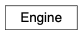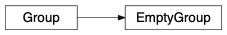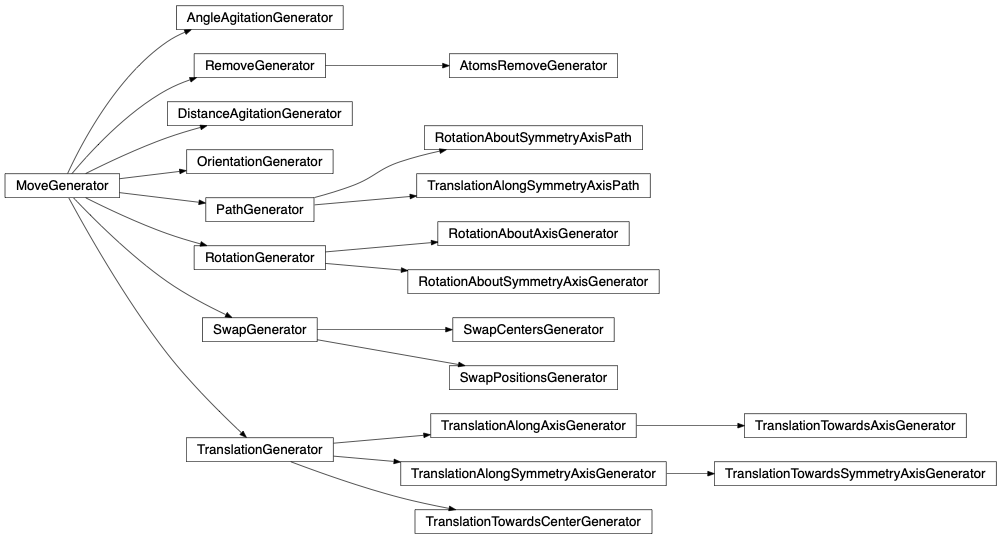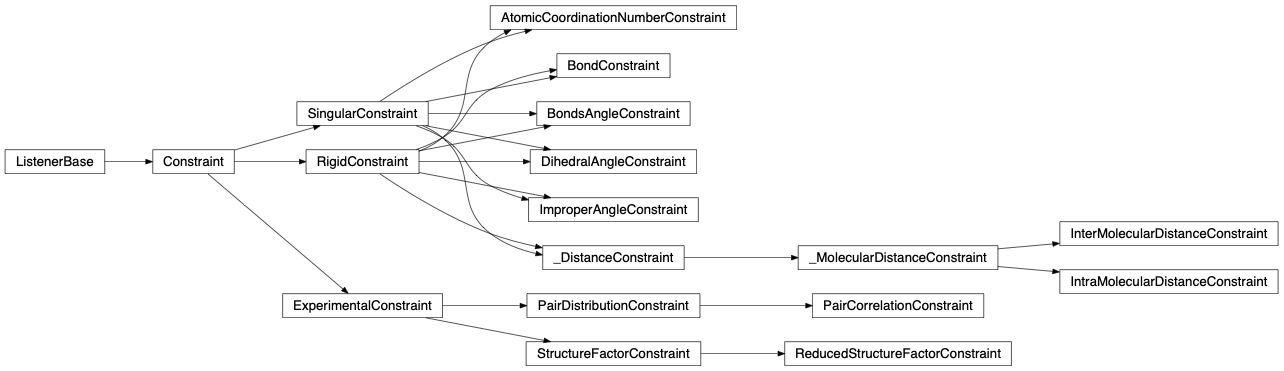Welcoming videos¶

 Molecular system fullrmc stochastic fitting simulation. Groups are set to molecules and smart moves are applied. Translations along symmetry axes, rotations about symmetry axes, etc. Atomic binary Nickel-Titanium shape memory alloy system phase transformation stochastic simulation. Random atomic translations are enough to reproduce short range ordering. But swapping atoms is necessary to fit long range atomic correlations. Molecular system mere atomic stochastic simulation. Covalent bond electron density polarization is modelled by allowing fullrmc to explore across energy low correlation barriers. Reverse Monte Carlo traditional fitting mode compared with fullrmc’s recursive selection with exploring. This video shows how from a potential point of view exploring allow to cross forbidden unlikely barriers and going out of local minimas.

Brief Description¶

Reverse Monte Carlo (RMC) is probably best known for its applications in condensed matter physics and solid state chemistry. fullrmc which stands for FUndamental Library Language for Reverse Monte Carlo is different than traditional RMC but a stochastic modelling method to solve an inverse problem whereby an atomic/molecular model is adjusted until its atoms position have the greatest consistency with a set of experimental data.

fullrmc is a python package with its core and calculation modules optimized and compiled in Cython. fullrmc is not a standard RMC package but it’s rather unique in its approach to stochastically solving an atomic or molecular structure. fullrmc’s Engine sub-module is the main module that contains the definition of ‘Engine’ which is the main and only class used to launch a stochastic fitting calculation. Engine reads only Protein Data Bank formatted atomic configuration ‘.pdb’ files and handles other definitions and attributes such as:

1. Group: Engine doesn’t understand atoms or molecules but group of atom indexes instead. A group is a set of atom indexes, allowing indexes redundancy within the same group definition. A Group instance can contain any list of indexes and as many atoms index as needed. Grouping atoms is essential to make clusters of atoms (residues, molecules, etc) evolve and move together. A group of a single atom index can be used to make a single atom move separately from the others. Engine’s ‘groups’ attribute is a simple list of group instances containing all the desired and defined groups that one wants to move.
2. Group selector: Engine requires a GroupSelector instance which is the artist that selects a group from the engine’s groups list at every engine runtime step. Among other properties, depending on which group selector is used by the engine, a GroupSelector can allow weighting which means selecting groups more or less frequently than the others, it can also allow selection recurrence and refinement of a single group, ordered and random selection is also possible.
3. Move generator: Every group instance has its own MoveGenerator. Therefore every group of atoms when selected by the engine’s group selector at the engine’s runtime can perform a customizable and different kind of moves.
4. Constraint: A constraint is a rule that controls certain aspect of the configuration upon moving groups. Engine’s ‘constraints’ attribute is a list of all defined and used constraint instances, it is the judge that controls the evolution of the system by accepting or rejecting the move of a group. If engine’s constraints list is empty and contains no constraint definition, this will result in accepting all the generated moves.

Tetrahydrofuran simple example yet complete and straight to the point¶

## Tetrahydrofuran (THF) molecule sketch
##
##              O
##   H41      /   \      H11
##      \  /         \  /
## H42-- C4    THF    C1 --H12
##        \  MOLECULE /
##         \         /
##   H31-- C3-------C2 --H21
##        /         \
##     H32            H22
##

#   #####################################################################################   #
#   ############################### IMPORT WHAT IS NEEDED ###############################   #
import os
import numpy as np
from fullrmc.Engine import Engine
from fullrmc.Constraints.PairDistributionConstraints import PairDistributionConstraint
from fullrmc.Constraints.DistanceConstraints import InterMolecularDistanceConstraint
from fullrmc.Constraints.BondConstraints import BondConstraint
from fullrmc.Constraints.AngleConstraints import BondsAngleConstraint
from fullrmc.Constraints.ImproperAngleConstraints import ImproperAngleConstraint
from fullrmc.Core.MoveGenerator import MoveGeneratorCollector
from fullrmc.Generators.Translations import TranslationGenerator, TranslationAlongSymmetryAxisGenerator
from fullrmc.Generators.Rotations import RotationGenerator, RotationAboutSymmetryAxisGenerator

#   #####################################################################################   #
#   ############################# DECLARE USEFUL VARIABLES ##############################   #
pdfData      = "thf_pdf.exp"
pdbStructure = "thf.pdb"
enginePath   = "thf_engine.rmc"

#   #####################################################################################   #
#   ############################## CREATE STOCHASTIC ENGINE #############################   #
ENGINE = Engine(path=None)
# if engine is not created and saved
if not ENGINE.is_engine(enginePath)
# create and initialize engine
ENGINE = Engine(path=enginePath, freshStart=True)
ENGINE.set_pdb(pdbFileName)

# re-set structure boundary conditions
ENGINE.set_boundary_conditions(np.array([48.860,0,0, 0,48.860,0, 0,0,48.860]))

# create and add pair distribution constraint to the engine
PDF_CONSTRAINT = PairDistributionConstraint(experimentalData=pdfData, weighting="atomicNumber")

# create and add intermolecular distances constraint to the engine
EMD_CONSTRAINT = InterMolecularDistanceConstraint()

# create and add bonds constraint to the engine
B_CONSTRAINT = BondConstraint()
B_CONSTRAINT.create_bonds_by_definition( bondsDefinition={"THF": [('O' ,'C1' , 1.20, 1.70),
('O' ,'C4' , 1.20, 1.70),
('C1','C2' , 1.25, 1.90),
('C2','C3' , 1.25, 1.90),
('C3','C4' , 1.25, 1.90),
('C1','H11', 0.88, 1.16),('C1','H12', 0.88, 1.16),
('C2','H21', 0.88, 1.16),('C2','H22', 0.88, 1.16),
('C3','H31', 0.88, 1.16),('C3','H32', 0.88, 1.16),
('C4','H41', 0.88, 1.16),('C4','H42', 0.88, 1.16)] })

# create and add angles constraint to the engine
BA_CONSTRAINT = BondsAngleConstraint()
BA_CONSTRAINT.create_angles_by_definition( anglesDefinition={"THF": [ ('O'  ,'C1' ,'C4' , 105, 125),
('C1' ,'O'  ,'C2' , 100, 120),
('C4' ,'O'  ,'C3' , 100, 120),
('C2' ,'C1' ,'C3' , 95 , 115),
('C3' ,'C2' ,'C4' , 95 , 115),
# H-C-H angle
('C1' ,'H11','H12', 98 , 118),
('C2' ,'H21','H22', 98 , 118),
('C3' ,'H31','H32', 98 , 118),
('C4' ,'H41','H42', 98 , 118),
# H-C-O angle
('C1' ,'H11','O'  , 100, 120),
('C1' ,'H12','O'  , 100, 120),
('C4' ,'H41','O'  , 100, 120),
('C4' ,'H42','O'  , 100, 120),
# H-C-C
('C1' ,'H11','C2' , 103, 123),
('C1' ,'H12','C2' , 103, 123),
('C2' ,'H21','C1' , 103, 123),
('C2' ,'H21','C3' , 103, 123),
('C2' ,'H22','C1' , 103, 123),
('C2' ,'H22','C3' , 103, 123),
('C3' ,'H31','C2' , 103, 123),
('C3' ,'H31','C4' , 103, 123),
('C3' ,'H32','C2' , 103, 123),
('C3' ,'H32','C4' , 103, 123),
('C4' ,'H41','C3' , 103, 123),
('C4' ,'H42','C3' , 103, 123) ] })

# create and add improper angles constraint to the engine keeping THF molecules' atoms in the plane
IA_CONSTRAINT = ImproperAngleConstraint()
IA_CONSTRAINT.create_angles_by_definition( anglesDefinition={"THF": [ ('C2','O','C1','C4', -15, 15),
('C3','O','C1','C4', -15, 15) ] })

# initialize constraints data
ENGINE.initialize_used_constraints()

# save engine
ENGINE.save()
# if engine is created and saved, it can be simply loaded.
else:
# unpack constraints before fitting in case tweaking is needed
PDF_CONSTRAINT, EMD_CONSTRAINT, B_CONSTRAINT, BA_CONSTRAINT, IA_CONSTRAINT = ENGINE.constraints

#   #####################################################################################   #
#   ########################### RUN ATOMIC STOCHASTIC FITTING ###########################   #
# set groups as atoms. By default when the engine is constructed, all groups are single atoms.
ENGINE.set_groups_as_atoms()
ENGINE.run(numberOfSteps=100000, saveFrequency=1000)

#   #####################################################################################   #
#   ########################## RUN MOLECULAR STOCHASTIC FITTING #########################   #
## set groups as molecules instead of atoms
ENGINE.set_groups_as_molecules()
# set moves generators to all groups as a collection of random translation and rotation
for g in ENGINE.groups:
mg = MoveGeneratorCollector(collection=[TranslationGenerator(),RotationGenerator()], randomize=True)
g.set_move_generator( mg )
## Uncomment to use any of the following moves generators instead of the earlier collector
## Also other moves generators can be used to achieve a better fit for instance:
#[g.set_move_generator(TranslationAlongSymmetryAxisGenerator(axis=0)) for g in ENGINE.groups]
#[g.set_move_generator(TranslationAlongSymmetryAxisGenerator(axis=1)) for g in ENGINE.groups]
#[g.set_move_generator(TranslationAlongSymmetryAxisGenerator(axis=2)) for g in ENGINE.groups]
#[g.set_move_generator(RotationAboutSymmetryAxisGenerator(axis=0))    for g in ENGINE.groups]
#[g.set_move_generator(RotationAboutSymmetryAxisGenerator(axis=1))    for g in ENGINE.groups]
#[g.set_move_generator(RotationAboutSymmetryAxisGenerator(axis=2))    for g in ENGINE.groups]
## Molecular constraints are not necessary any more because groups are set to molecules.
## At every engine step a whole molecule is rotate or translated therefore its internal
## distances and properties are safe from any changes. At any time constraints can be
## turn on again using the same method with a True flag. e.g. B_CONSTRAINT.set_used(True)
B_CONSTRAINT.set_used(False)
BA_CONSTRAINT.set_used(False)
IA_CONSTRAINT.set_used(False)
## run engine and perform stochastic fitting on molecules
ENGINE.run(numberOfSteps=100000, saveFrequency=1000)

#   #####################################################################################   #
#   ################################## PLOT CONSTRAINTS #################################   #
PDF_CONSTRAINT.plot(show=False)
EMD_CONSTRAINT.plot(show=False)
B_CONSTRAINT.plot(lineWidth=2, nbins=20,  split='element', show=False)
BA_CONSTRAINT.plot(lineWidth=2, nbins=20, split='element', show=False)
IA_CONSTRAINT.plot(lineWidth=2, nbins=20, split='element', show=True )

The result shown in the figures herein is obtained by running fullrmc Engine for several hours on molecular groups. Position optimization is achieved by using a RecursiveGroupSelector to refine every selected group position and alternating groups move generators. RotationAboutSymmetryAxisGenerator is used to fit the ring orientation, then TranslationAlongSymmetryAxisGenerator is used to translate molecules along meaningful directions. At the end, reset groups to single atom index and RandomSelector is used to select groups randomly. the Engine is run for additional several hours to refine atoms positions separately.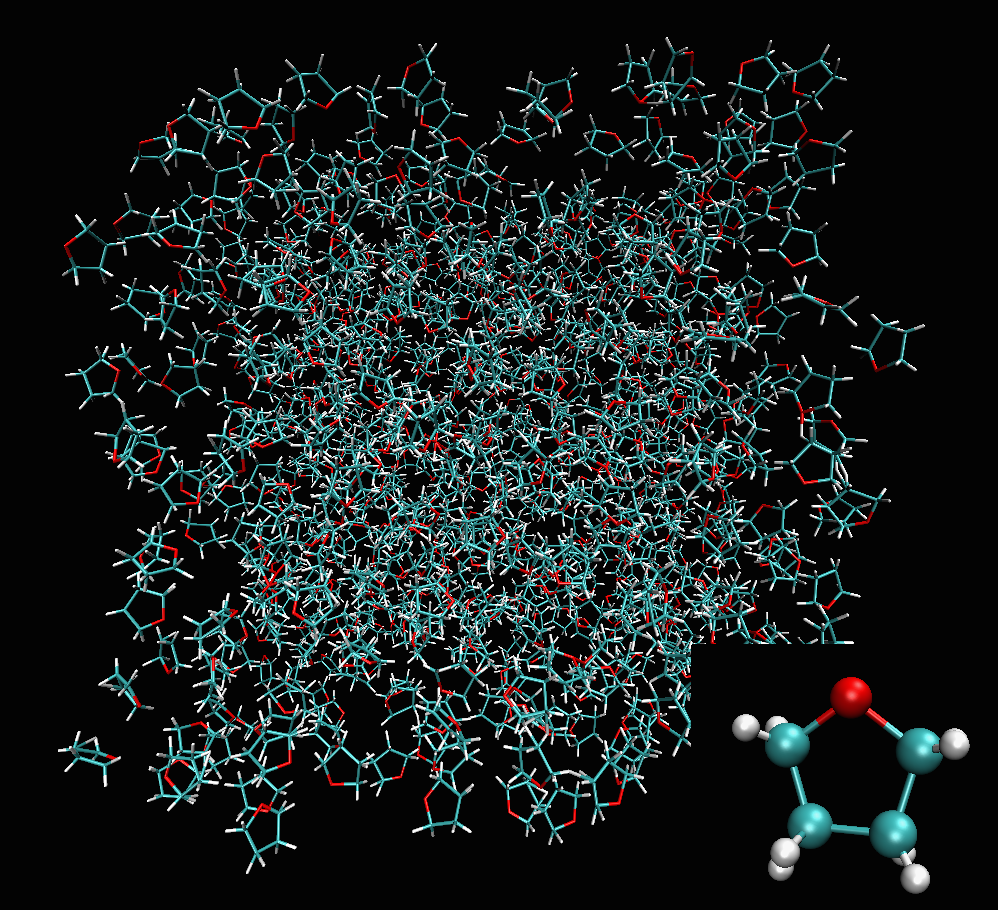a) Structure containing 800 Tetrahydrofuran randomly generated.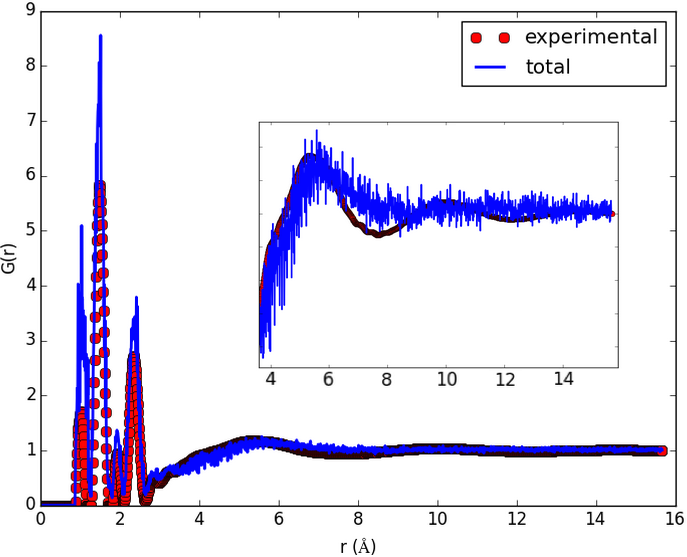b) Initial pair distribution function calculated before any fitting.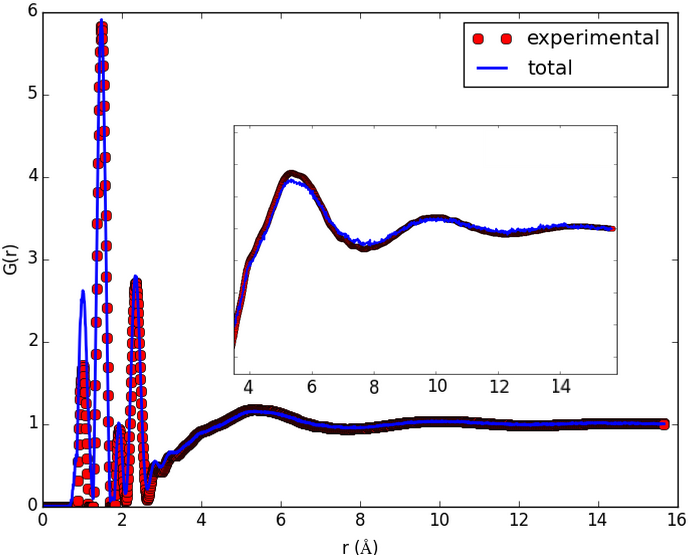c) pair distribution function calculated after about 20 hours of Engine runtime.

Sub-modules¶

Engine is fullrmc’s main module. It contains ‘Engine’ the main class of fullrmc which is the stochastic artist. The engine class takes only Protein Data Bank formatted files ‘.pdb’ as atomic/molecular input structure. It handles and fits simultaneously many experimental data while controlling the evolution of the system using user-defined molecular and atomistic constraints such as bond-length, bond-angles, dihedral angles, inter-molecular-distances, etc.

class fullrmc.Engine.Engine(path=None, frames=None, logFile=None, freshStart=False)

Bases: object

fulrmc’s engine, is used to launch a stochastic modelling which is different than traditional Reverse Monte Carlo (RMC). It has the capability to use and fit simultaneously multiple sets of experimental data. One can also define constraints such as distances, bonds length, angles and many others.

Parameters: path (None, string): Engine repository (directory) path to save the engine. If None is given path will be set when saving the engine using Engine.save method. If a non-empty directory is found at the given path an error will be raised unless freshStart flag attribute is set to True. frames (None, list): List of frames name. Frames are used to store fitting data. Multiple frames can be used to create a fitting story or to fit multiple structures simultaneously. Also multiple frames can be used to launch multiple simulations at the same time and merge structures at some predefined merging frequency. If None is given, a single frame ‘0’ is initialized automatically. logFile (None, string): Logging file basename. A logging file full name will be the given logFile appended ‘.log’ extension automatically. If None is given, logFile is left unchanged. freshStart (boolean): Whether to remove any existing fullrmc engine at the given path if found. If set to False, an error will be raise if a fullrmc engine or a non-empty directory is found at the given path.
# import engine
from fullrmc.Engine import Engine

# create engine
ENGINE = Engine(path='my_engine.rmc')

# set pdb file
ENGINE.set_pdb(pdbFileName)

# Add constraints ...
# Re-define groups if needed ...
# Re-define groups selector if needed ...
# Re-define moves generators if needed ...

# save engine
ENGINE.save()

# run engine for 10000 steps and save only at the end
ENGINE.run(numberOfSteps=10000, saveFrequency=10000, savePath="system.rmc")
info

Engine’s information (version, id) tuple.

frames

Engine’s frames list copy.

usedFrame

Engine’s frame in use.

lastSelectedGroupIndex

The last moved group instance index in groups list.

lastSelectedGroup

The last moved group instance.

lastSelectedAtomsIndex

The last moved atoms index.

state

Engine’s state.

generated

Number of generated moves.

removed

Removed atoms tuple (tried, accepted, ratio)

tried

Number of tried moves.

accepted

Number of accepted moves.

tolerated

Number of tolerated steps in spite of increasing totalStandardError

tolerance

Tolerance in percent.

groups

Engine’s defined groups list.

pdb

Engine’s pdbParser instance.

boundaryConditions

Engine’s boundaryConditions instance.

isPBC

Whether boundaryConditions are periodic.

isIBC

Whether boundaryConditions are infinte.

basisVectors

The boundary conditions basis vectors in case of PeriodicBoundaries, None in case of InfiniteBoundaries.

reciprocalBasisVectors

The boundary conditions reciprocal basis vectors in case of PeriodicBoundaries, None in case of InfiniteBoundaries.

volume

The boundary conditions basis volume in case of PeriodicBoundaries, None in case of InfiniteBoundaries.

realCoordinates

The real coordinates of the current configuration.

boxCoordinates

The box coordinates of the current configuration in case of PeriodicBoundaries. Similar to realCoordinates in case of InfiniteBoundaries.

numberOfMolecules

Number of molecules.

moleculesIndex

Atoms molecule index list.

moleculesName

Atoms molecule name list.

elementsIndex

Atoms element index list indexing elements sorted set.

elements

Sorted set of all existing atom elements.

allElements

Atoms element list.

namesIndex

Atoms name index list indexing names sorted set

names

Sorted set of all existing atom names.

allNames

Atoms name list.

numberOfNames

Length of atoms name set.

numberOfAtoms

Number of atoms in the pdb.

numberOfAtomsPerName

Number of atoms per name dictionary.

numberOfElements

Number of different elements in the pdb.

numberOfAtomsPerElement

Number of atoms per element dictionary.

numberDensity

System’s number density computed as $$\rho_{0}=\frac{N}{V}$$ where N is the total number of atoms and V the volume of the system.

constraints

Copy list of all constraints instances.

groupSelector

Engine’s group selector instance.

totalStandardError

Engine’s last recorded totalStandardError of the current configuration.

get_original_data(name)

Get original data as initialized and parsed from pdb.

Parameters: name (string): Data name. value (object): Data value
is_engine(path, repo=False, mes=False)

Get whether a fullrmc engine is stored in the given path.

Parameters: path (string): The path to fetch. repo (boolean): Whether to return repository if an engine is found. Otherwise None is returned. mes (boolean): Whether to return explanatory message. result (boolean): The fetch result, True if engine is found False otherwise. repo (pyrep.Repository): The repository instance. This is returned only if ‘repo’ argument is set to True. message (string): The explanatory message. This is returned only if ‘mes’ argument is set to True.
save(path=None, copyFrames=True)

Save engine to disk.

Parameters: path (None, string): Repository path to save the engine. If path is None, engine’s path will be used. If path and engine’s path are both None, and error will be raised. copyFrames (boolean): If path is None, this argument is discarded. This argument sets whether to copy all frames data to the new repository path. If path is not None and this argument is False, Only used frame data will be copied and other frames will be discarded in new engine.

N.B. If path is given, it will automatically update engine’s path to point towards given path.

Load and return engine instance. None of the current engine attribute will be updated. must be used as the following:

# import engine
from fullrmc.Engine import Engine

# create engine
Parameters: path (string): Directory path to save the engine. engine (Engine): Engine instance.
set_log_file(logFile)

Set the log file basename.

Parameters: logFile (None, string): Logging file basename. A logging file full name will be the given logFile appended ‘.log’ extension automatically.
is_frame(frame)

Check whether a given frame exists.

Parameters: frame (string): Frame name. result (boolean): True if frame exists False otherwise.

Add a single or multiple frames to engine.

Parameters: frames (string, list): Frames name. It can be a string to add a single frame or a list of strings to add multiple frames.

Add a single frame to engine.

Parameters: frame (string): Frame name.
reinit_frame(frame)

Reset frame data to initial pdb coordinates.

Parameters: frame (string): The frame name to set.
set_used_frame(frame)

Switch engine frame.

Parameters: frame (string): The frame to switch to and use from now on.
delete_frame(frame)

Delete frame data from Engine as well as from system.

Parameters: frame (string): The frame to delete.
rename_frame(frame, newName)

Rename frame.

Parameters: frame (string): The frame to rename. newName (string): The new name.
export_pdb(path)

Export a pdb file of the last refined and saved configuration state.

Parameters: path (string): the pdb file path.
get_pdb()

Get a pdb instance of the last refined and save configuration state.

Returns: pdb (pdbParser): The pdb instance.
set_tolerance(tolerance)

Set engine’s runtime tolerance value.

Parameters: tolerance (number): The runtime tolerance parameters. It’s the percentage of allowed unsatisfactory ‘tried’ moves.
set_group_selector(selector)

Set engine’s group selector instance.

Parameters: selector (None, GroupSelector): The GroupSelector instance. If None is given, RandomSelector is set automatically.
clear_groups()

Clear all engine’s defined groups.

Add a group to engine’s groups list.

Parameters: g (Group, integer, list, set, tuple numpy.ndarray): Group instance, integer, list, tuple, set or numpy.ndarray of atoms index. broadcast (boolean): Whether to broadcast “update groups”. This is to be used interally only. Keep default value unless you know what you are doing.
set_groups(groups)

Set engine’s groups.

Parameters: groups (None, Group, list): A single Group instance or a list, tuple, set of any of Group instance, integer, list, set, tuple or numpy.ndarray of atoms index that will be set one by one by set_group method. If None is given, single atom groups of all atoms will be all automatically created which is the same as using set_groups_as_atoms method.

Add groups to engine.

Parameters: groups (Group, list): Group instance or list of groups, where every group must be a Group instance or a numpy.ndarray of atoms index of type numpy.int32.
set_groups_as_atoms()

Automatically set engine’s groups as single atom group for all atoms.

set_groups_as_molecules()

Automatically set engine’s groups indexes according to molecules indexes.

set_pdb(pdb, boundaryConditions=None, names=None, elements=None, moleculesIndex=None, moleculesName=None)

Set used frame pdb configuration. Engine and constraints data will be automatically reset but not constraints definitions. If pdb was already set and this is a resetting of a different atomic configuration, with different elements or atomic order, or different size and number of atoms, constraints definitions must be reset manually. In general, their is no point in changing the atomic configuration of a completely different atomic nature. It is advisable to create a new engine from scratch or redefining all constraints definitions.

Parameters: pdb (pdbParser, string): the configuration pdb as a pdbParser instance or a path string to a pdb file. boundaryConditions (None, InfiniteBoundaries, PeriodicBoundaries, numpy.ndarray, number): The configuration’s boundary conditions. If None, boundaryConditions will be parsed from pdb if existing otherwise, InfiniteBoundaries with no periodic boundaries will be set. If numpy.ndarray is given, it must be pass-able to a PeriodicBoundaries instance. Normally any real numpy.ndarray of shape (1,), (3,1), (9,1), (3,3) is allowed. If number is given, it’s like a numpy.ndarray of shape (1,), it is assumed as a cubic box of box length equal to number. names (None, list): Atoms names list. If None is given, names will be calculated automatically by parsing pdb instance. elements (None, list): Atoms elements list. If None is given, elements will be calculated automatically by parsing pdb instance. moleculesIndex (None, list, numpy.ndarray): Molecules index list. Must have the length of number of atoms. If None is given, moleculesIndex will be calculated automatically by parsing pdb instance. moleculesName (None, list): Molecules name list. Must have the length of the number of atoms. If None is given, it is automatically generated as the pdb residues name.
set_boundary_conditions(boundaryConditions)

Sets the configuration’s boundary conditions. Any type of periodic boundary conditions is allowed and not restricted to cubic. Engine and constraints data will be automatically reset.

Parameters: boundaryConditions (None, InfiniteBoundaries, PeriodicBoundaries, numpy.ndarray, number): The configuration’s boundary conditions. If None, InfiniteBoundaries with no periodic boundaries will be set. If numpy.ndarray is given, it must be pass-able to a PeriodicBoundaries instance. Normally any real numpy.ndarray of shape (1,), (3,1), (9,1), (3,3) is allowed. If number is given, it’s like a numpy.ndarray of shape (1,), it is assumed as a cubic box of box length equal to number.
set_number_density(numberDensity)

Sets system’s number density. This is used to correct system’s volume. It can only be used with InfiniteBoundaries.

Parameters: numberDensity (number): Number density value that should be bigger than zero.
set_molecules_index(moleculesIndex=None, moleculesName=None)

Set moleculesIndex list, assigning each atom to a molecule.

Parameters: moleculesIndex (None, list, numpy.ndarray): Molecules index list. Must have the length of the number of atoms. If None is given, moleculesIndex will be calculated automatically by parsing pdb instance. moleculesName (None, list): Molecules name list. Must have the length of the number of atoms. If None is given, it will be automatically generated as the pdb residues name.
set_elements_index(elements=None)

Set elements and elementsIndex lists, assigning a type element to each atom.

Parameters: elements (None, list): Elements list. Must have the length of the number of atoms. If None is given, elements will be calculated automatically by parsing pdb instance.
set_names_index(names=None)

Set names and namesIndex list, assigning a name to each atom.

Parameters: #. names (None, list): The names list. If None is given, names will be generated automatically by parsing pdbParser instance.
visualize(commands=None, foldIntoBox=False, boxToCenter=False, boxWidth=2, boxStyle='solid', boxColor='yellow', bgColor='black', displayParams=None, representationParams='Lines', otherParams=None)

Visualize the last configuration using pdbParser visualize_vmd method.

Parameters: commands (None, list, tuple): List of commands to pass upon calling vmd. foldIntoBox (boolean): Whether to fold all atoms into PeriodicBoundaries box before visualization. If boundary conditions are InfiniteBoundaries then nothing will be done. boxToCenter (boolean): Translate box center to atom coordinates center. boxWidth (number): Visualize the simulation box by giving the lines width. If 0 or boundary conditions are InfiniteBoundaries then nothing is visualized. boxStyle (str): The box line style, it can be either solid or dashed. If boundary conditions are InfiniteBoundaries then nothing will be done. boxColor (str): Choose the simulation box color. If boundary conditions are InfiniteBoundaries then nothing will be done. available colors are: blue, red, gray, orange, yellow, tan, silver, green, white, pink, cyan, purple, lime, mauve, ochre, iceblue, black, yellow2, yellow3, green2, green3, cyan2, cyan3, blue2, blue3, violet, violet2, magenta, magenta2, red2, red3, orange2, orange3. bgColor (str): Set visualization background color. displayParams(None, dict): Set display parameters. If None is given, default parameters will be applied. If dictionary is given, the following keys can be used. ‘depth cueing’ (default True): Set the depth cueing flag. ‘cue density’ (default 0.1): Set the depth density. ‘cue mode’ (default ‘Exp’): Set the depth mode among ‘linear’, ‘Exp’ and ‘Exp2’. representationParams(str): Set representation method among the following: Lines, Bonds, DynamicBonds, HBonds, Points, VDW, CPK, Licorice, Beads, Dotted, Solvent. Add parameters accordingly if needed like the following. Points representation accept only size parameter e.g. ‘Points 5’ CPK representation can accept respectively 4 parameters as the following ‘Sphere Scale’, ‘Bond Radius’, ‘Sphere Resolution’, ‘Bond Resolution’ e.g. ‘CPK 1.0 0.2 50 50’ VDW representation can accept respectively 2 parameters as the following ‘Sphere Scale’, ‘Sphere Resolution’ e.g. ‘VDW 0.7 100’ otherParams(None, list, set, tuple): Any other parameters in a form of a list of strings. e.g. [‘display resize 700 700’, ‘rotate x to 45’, ‘scale to 0.02’, ‘axes location off’]

Add constraints to the engine.

Parameters: constraints (Constraint, list, set, tuple): A constraint instance or list of constraints instances
remove_constraints(constraints)

Remove constraints from engine list of constraints.

Parameters: constraints (Constraint, list, set, tuple): A constraint instance or list of constraints instances
reset_constraints()

Reset constraints flags.

reset_engine()

Re-initialize engine and resets constraints flags and data.

compute_total_standard_error(constraints, current='standardError')

Compute the total standard error as the sum of all constraints’ standard error.

$\chi^{2} = \sum \limits_{i}^{N} (\frac{stdErr_{i}}{variance_{i}})^{2}$

Where:

$$variance_{i}$$ is the variance value of the constraint i.

$$stdErr_{i}$$ the standard error of the constraint i defined as $$\sum \limits_{j}^{points} (target_{i,j}-computed_{i,j})^{2} = (Y_{i,j}-F(X_{i,j}))^{2}$$

Parameters: constraints (list): All constraints used to calculate total totalStandardError. current (str): which standard error to use. can be anything like standardError, afterMoveStandardError or amputatedStandardError, etc. totalStandardError (list): The computed total total standard error.
set_total_standard_error()

Compute and set engine’s total totalStandardError of active constraints.

get_used_constraints(sortConstraints=False)

Parses all engine’s constraints and returns different lists of the active (used) ones.

Parameters: sortConstraints (boolean): Whether to sort used constraints according to their computation cost property. This is can minimize computations and enhance performance by computing less costly constraints first. usedConstraints (list): All types of active constraints instances that are used at engine’s runtime. constraints (list): All active constraints instance among usedConstraints list that will contribute to engine’s totalStandardError. RigidConstraint (list): All active RigidConstraint constraints instance among usedConstraints list that won’t contribute engine’s totalStandardError.
initialize_used_constraints(force=False, sortConstraints=False)

Calls get_used_constraints method, re-initializes constraints when needed and return them all.

Parameters: force (boolean): Whether to force initializing constraints regardless of their state. sortConstraints (boolean): Whether to sort used constraints according to their computation cost property. This is can minimize computations and enhance performance by computing less costly constraints first. usedConstraints (list): All types of active constraints instances that are used at engine’s runtime. constraints (list): All active constraints instance among usedConstraints list that will contribute to engine’s totalStandardError. RigidConstraint (list): All active RigidConstraint constraints instance among usedConstraints list that won’t contribute engine’s totalStandardError.
run(numberOfSteps=100000, sortConstraints=True, saveFrequency=1000, frame=None, xyzFrequency=None, xyzPath='trajectory.xyz', restartPdb='restart.pdb', ncores=None)

Run stochastic fitting engine.

Parameters: numberOfSteps (integer): The number of steps to run. sortConstraints (boolean): Whether to sort used constraints according to their computation cost property. This is can minimize computations and enhance performance by computing less costly constraints first. saveFrequency (integer): Save engine every saveFrequency steps. Save will be omitted if no moves are accepted. xyzFrequency (None, integer): Save coordinates to .xyz file every xyzFrequency steps regardless if totalStandardError has decreased or not. If None is given, no .xyz file will be generated. xyzPath (string): Save coordinates to .xyz file. restartPdb (None, string): Export a pdb file of the last configuration at the end of the run. If None is given, no pdb file will be exported. If string is given, it should be the full path of the pdb file. ncores (None, integer): set the number of cores to use. If None is given, ncores will be set automatically to 1. This argument is only effective if fullrmc is compiled with openmp.
fullrmc.Engine.generate_random_float()

random() -> x in the interval [0, 1).

This module provides all the global types, variables and some general methods that fullrmc needs.

Types:¶

1. INT_TYPE: The integer data type adopted by fullrmc.
2. FLOAT_TYPE: The floating data type adopted by fullrmc.

Variables:¶

1. PRECISION: The floating data type precision variable.
2. FLOAT_PLUS_INFINITY: The floating data type maximum possible number.
3. FLOAT_MINUS_INFINITY: The floating data type minimum possible number.
4. INT_PLUS_INFINITY: The integer data type maximum possible number.
5. INT_MINUS_INFINITY: The integer data type minimum possible number.
6. PI: pi the ratio of a circle’s circumference to its diameter.
class fullrmc.Globals.Logger(*args, **kwargs)

Bases: pysimplelog.SimpleLog.SingleLogger

custom_init()
fixed(message)

alias to message at fixed level

accepted(message)

alias to message at move accepted level

rejected(message)

alias to message at move rejected level

nottried(message)

alias to message at move not tried level

saved(message)

alias to message at save engine level

impl(message)

alias to message at implement engine level

implement(message)

alias to message at usage engine level

usage(message)

alias to message at usage engine level